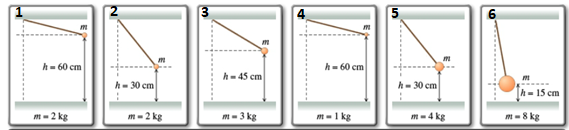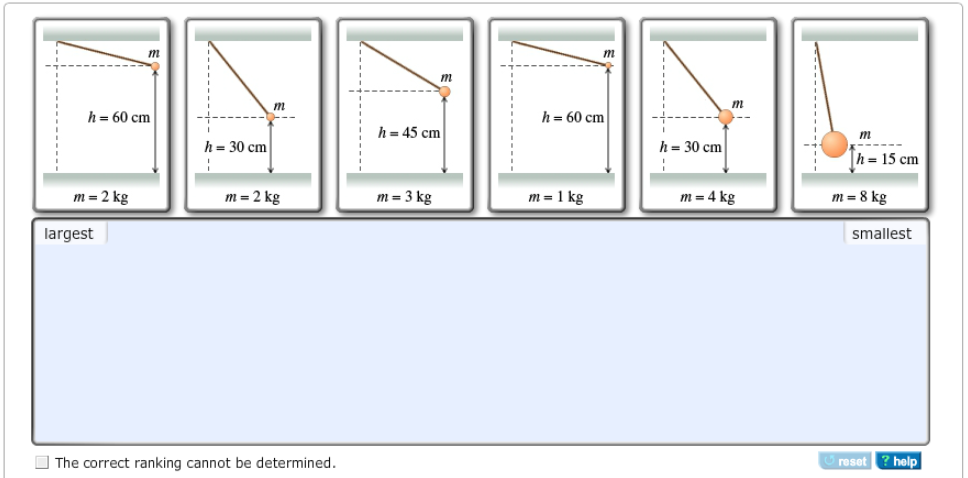# Problem: Rank each pendulum on the basis of the maximum kinetic energy it attains after release. Rank from largest to smallest. To rank items as equivalent, overlap them.

###### FREE Expert Solution

Gravitational potential energy is given by:

$\overline{){\mathbf{U}}{\mathbf{=}}{\mathbf{m}}{\mathbf{g}}{\mathbf{h}}}$From the conservation of energy, the maximum kinetic energy is equal to the potential energy of the system.

86% (115 ratings)###### Problem Details

Rank each pendulum on the basis of the maximum kinetic energy it attains after release. Rank from largest to smallest. To rank items as equivalent, overlap them.